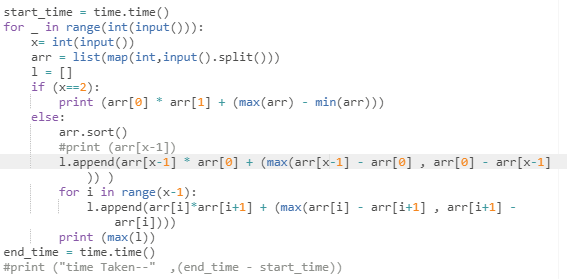# MVALUE - Editorial

But how you make sure that a and b are distinct elements? Please explain @iscsi
example test case:-
1
4
-1 -1 10 10

a and b should be distinct?

yes, what about that condition …?
no mention of that condition in the editorial
I was using set to remove duplicate elements but I got WA only :[

I did watch the video but still can’t understand where my code’s going wrong

In python:You can check my solution using set.
https://www.codechef.com/viewsolution/43248600

m=max(m,(arr[n-1]*arr[n-2])+((arr[n-1]-arr[n-2])));
This is the wrong bit, when you know that this is for negative numbers, you should try and put some into it.
eg, for -6, -7 :
→ (-7 * -6) + (-7 - (-6)) = 42 + (-1) // your solution
whereas,
→ (-7 * -6) + (-6 - (-7)) = 42 + (+1), which is clearly greater
so all you need to do is make it (arr[n-2]-arr[n-1]);

Thanks@sanjeev0007 a,b can be theh same value but they have to be a different elements in the array, e.g if the array has two 10 than a,b both can be 10, in your example you can choose the 3rd, and the 4th item of the array.

``````#include<bits/stdc++.h>
#define int long long int

using namespace std;

void solve()
{
int n;cin>>n;

int arr[n];

for(int i=0;i<n;i++)
{
cin>>arr[i];
}

sort(arr,arr+n);

int ans = arr[n-1]*arr[n-2]+arr[n-1]-arr[n-2];

cout<<ans<<endl;

}

signed main()
{
ios_base::sync_with_stdio(false);
cin.tie(0);
cout.tie(0);

int t;
cin>>t;
while(t--)solve();
return 0;
}
``````

can anyone tell me why is this getting wa

why it is given a and b are distinct elements, i thought if 2 same elements are present in array then i have to chose the smaller one apart from same number.

numbers in array are negative also and it may happen that two negative number produce product greater than 2 positive number.

import java.util.;
import java.lang.
;
import java.io.*;
import java.math.BigInteger;

/* Name of the class has to be “Main” only if the class is public. */
class Codechef{

``````public static void main (String[] args) throws java.lang.Exception{

while(t-->0){
long arr[]=new long[n];
for(int i=0;i<n;i++){
arr[i]=Long.parseLong(t1[i]);
}
Arrays.sort(arr);
long a=arr[n-1];
long b=arr[n-2];
long x=arr;
long y=arr;
long sum=Math.max(a*b+a-b,x*y+x-y);
System.out.println(sum);
}
``````

}
}

1 Like

or just sort athe array by Arrays.sort() and then take the same.

absolutely right ,have to be different element but can be same value .

I totally forgot the negative side of numbers!, my bad.

Why so?

Only reason I marked it easy-med because the idea to rewrite expression is what I believed many would miss. Otherwise I’d only mark it easy if (a-1)*(b+1) was given.

Do you mean to say that this problem has the same difficulty as this problem or am I mistaken somewhere?
Also, I don’t think that VSTRING was anyways easier than this problem. At least the numbers don’t seem to agree.

Sorry If there’s different criteria for assigning these difficulties that I’m not aware of.

1 Like

where a and b are two are 2 distinct elements of the array.

This was quite misleading. I assume 2 distinct elements by magnitude and got W.A.
However nice question and video editorial is too good.

I found problem VSTRING much easier when compared to problem MVALUE. Because as soon as I read the problem VSTRING, I know what to do and had proof for the same within a minute. But that was not the same for MVALUE as it took me time to prove my solution.

Coming to the difficulty tag for the problem, I think the targeted audience also affects the difficulty tag. As in this problem MVALUE, the targeted audience was Division 3 whereas, in problem INDEP, the targeted audience was different.